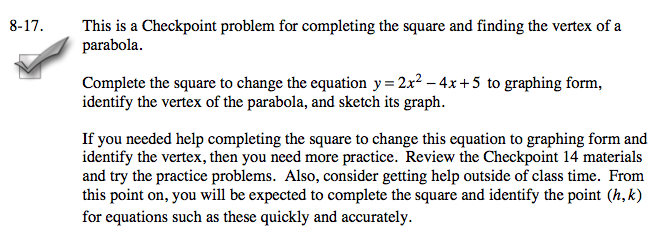### Home > A2C > Chapter 8 > Lesson 8.1.2 > Problem8-17

8-17.

This is a Checkpoint problem for completing the square and finding the vertex of a parabola.Homework Help ✎

Complete the square to change the equation y = 2x2 − 4x + 5 to graphing form, identify the vertex of the parabola, and sketch its graph.

If you needed help completing the square to change this equation to graphing form and identify the vertex, then you need more practice. Review the Checkpoint 14 materials and try the practice problems. Also, consider getting help outside of class time. From this point on, you will be expected to complete the square and identify the point (h, k) for equations such as these quickly and accurately.Answers and extra practice are located in the back of your printed textbook or in the Reference Tab of your eBook.
If you have an eBook for A2C , login and then click the following link: Checkpoint 14 Answers and Practice